# 想在论文中画出漂亮的插图，介绍一个利器

## 如何在论文中画出漂亮的插图？校招没人要

（以下图片均引用自 Thumbnail gallery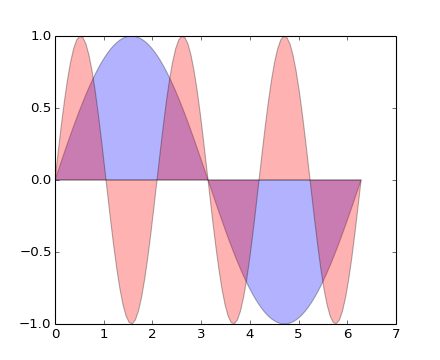``````plt.fill(x, y1, 'b', x, y2, 'r', alpha=0.3)
``````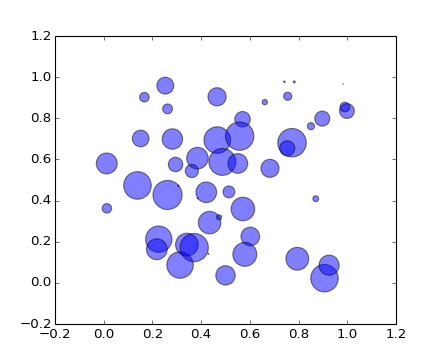``````plt.scatter(x, y, s=area, alpha=0.5)
``````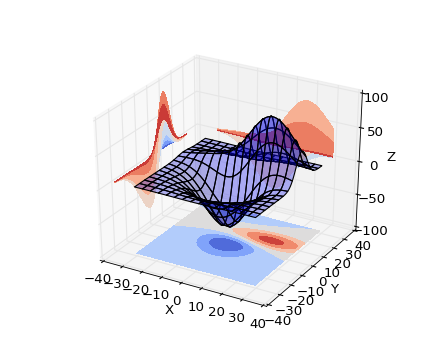``````ax.plot_surface(X, Y, Z, rstride=8, cstride=8, alpha=0.3)
cset = ax.contourf(X, Y, Z, zdir='z', offset=-100, cmap=cm.coolwarm)
cset = ax.contourf(X, Y, Z, zdir='x', offset=-40, cmap=cm.coolwarm)
cset = ax.contourf(X, Y, Z, zdir='y', offset=40, cmap=cm.coolwarm)
``````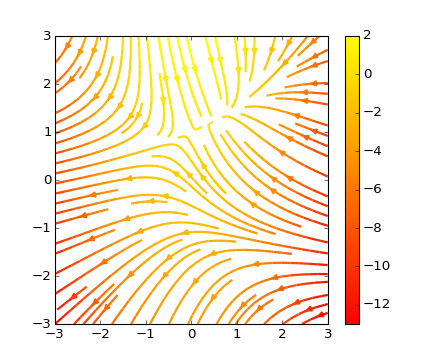``````plt.streamplot(X, Y, U, V, color=U, linewidth=2, cmap=plt.cm.autumn)
plt.colorbar()
``````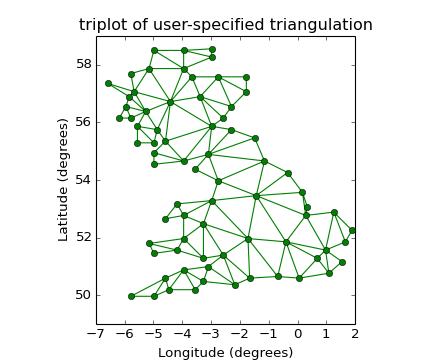``````plt.triplot(x, y, triangles, 'go-')
plt.title('triplot of user-specified triangulation')
plt.xlabel('Longitude (degrees)')
plt.ylabel('Latitude (degrees)')
``````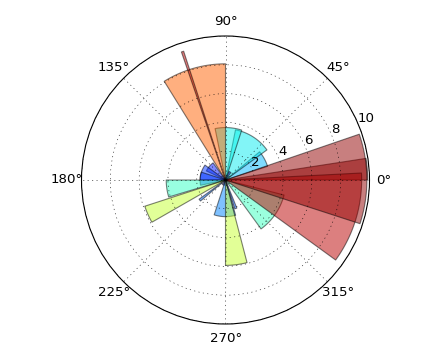``````ax = plt.subplot(111, polar=True)
bars = ax.bar(theta, radii, width=width, bottom=0.0)
``````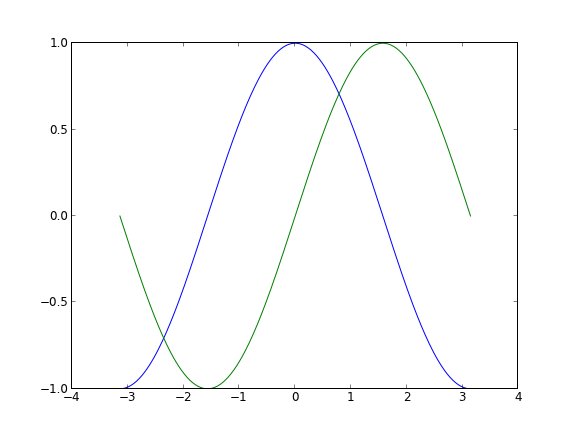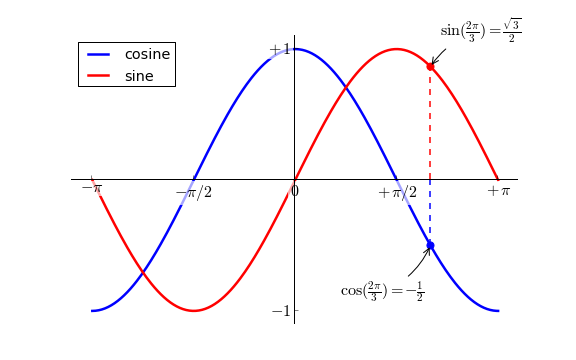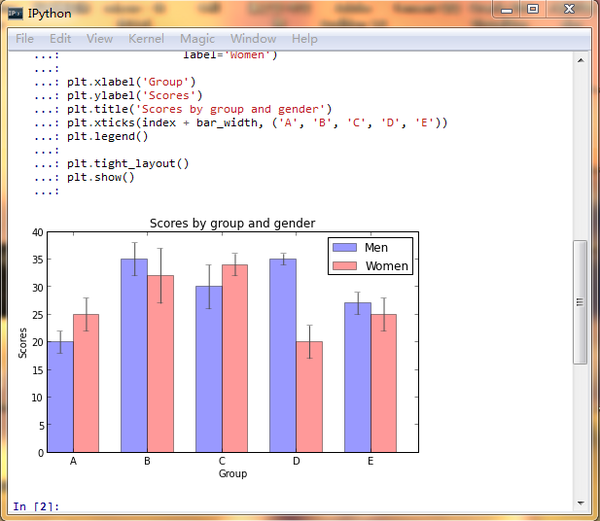（奉上教程一篇 Matplotlib Tutorial(译)

Update: 2013-9-18 18:04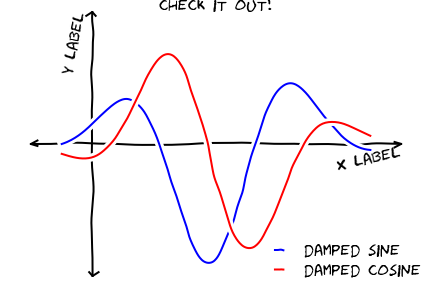（图片引用自网络）

Update: 2013-9-19 20:04

Update: 2013-10-1 23:45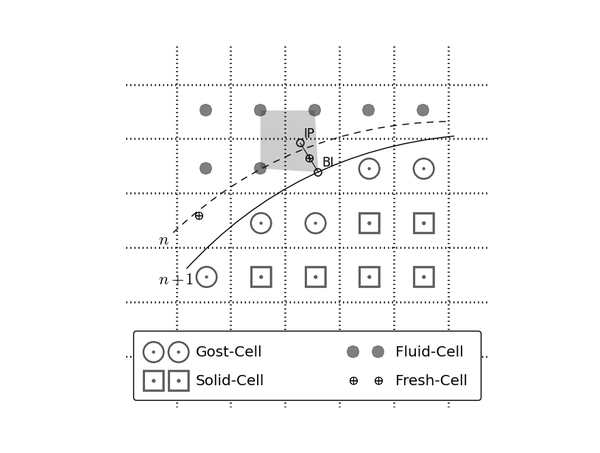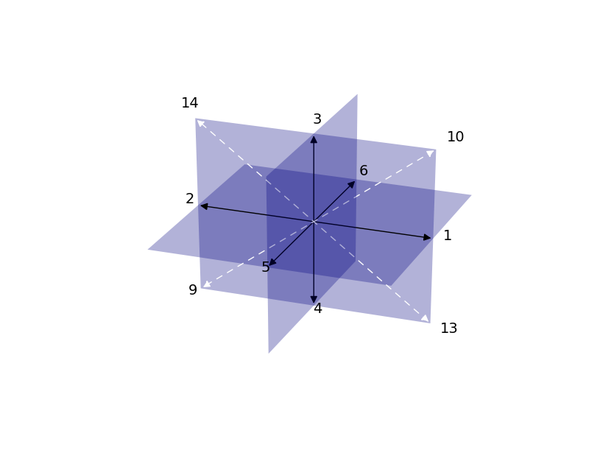2014.5.10 更新

（一下图片来源网络）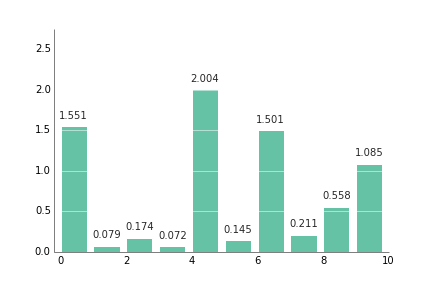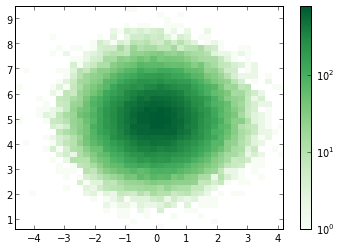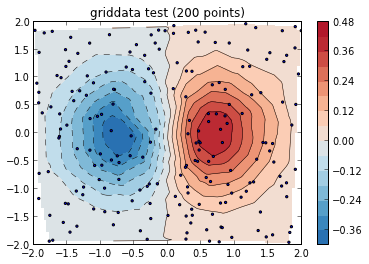``````cmap=brewer2mpl.get_map('RdBu', 'diverging', 8, reverse=True).mpl_colormap,
``````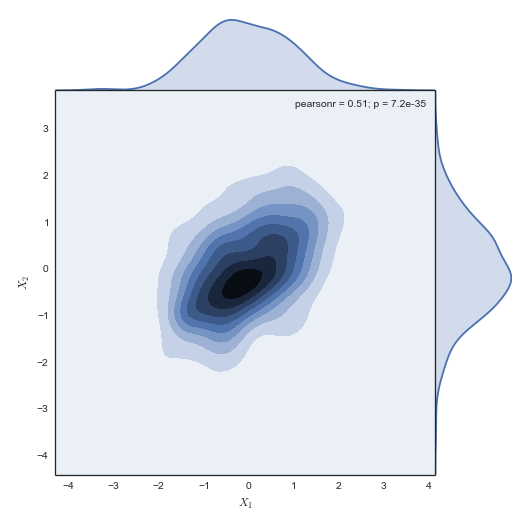``````g = sns.jointplot(x1, x2, kind="kde", size=7, space=0)
``````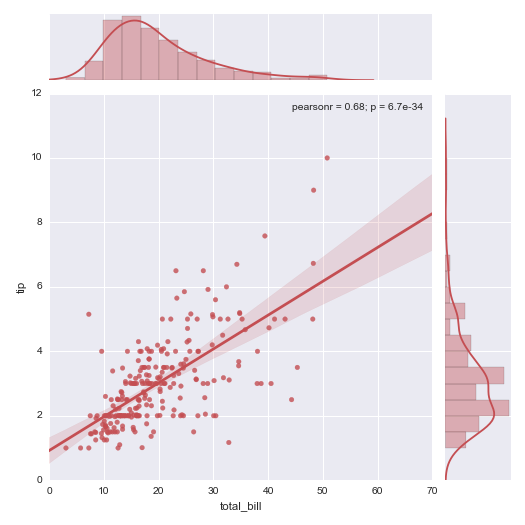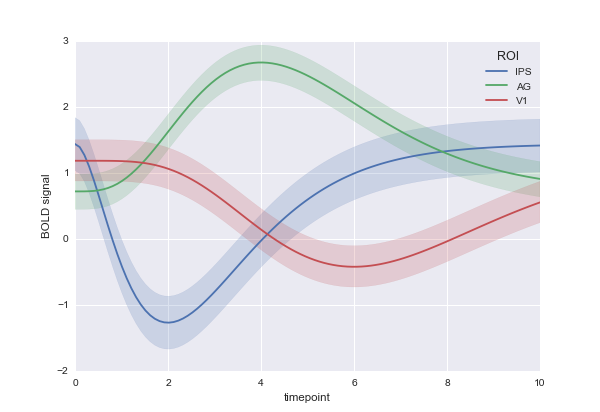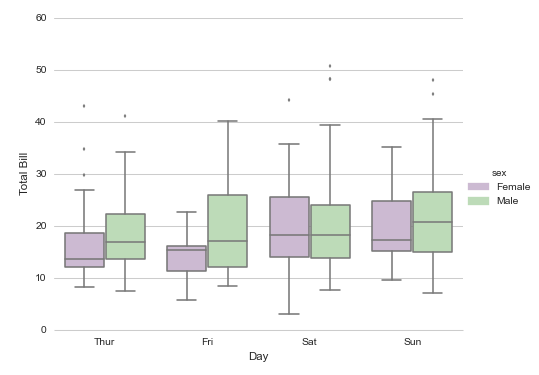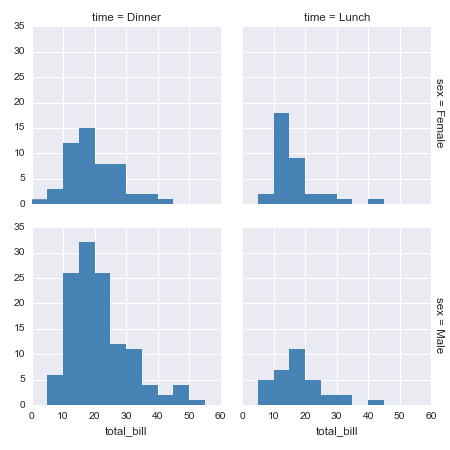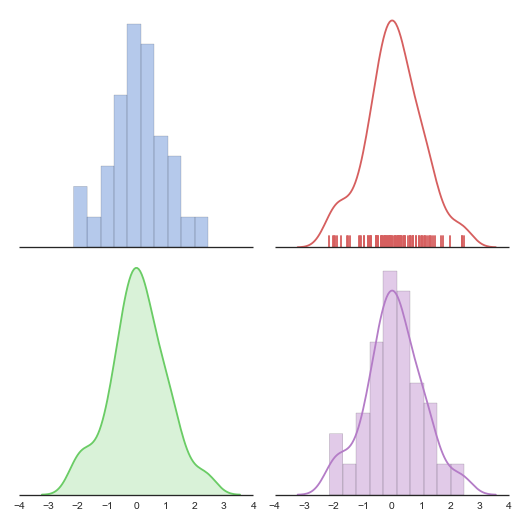## 扫描二维码下载知乎日报